Get inspired by the success stories of our students in IIT JAM MS, ISI  MStat, CMI MSc DS.  Learn More

# Cycles, Symmetry, and Counting | ISI MStat 2016 PSB | Problem 2This problem is a beautiful and elegant application of basic counting principles, symmetry and double counting principles in combinatorics. This is Problem 2 from ISI MStat 2016 PSB.

## Problem

Determine the average value of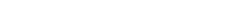taken over all permutations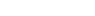of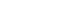.

## Solution

The problem may seem mind boggling at first, when you will even try to do it for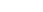, instead of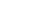.

But, in mathematics, symmetry is really intriguing. Let's see how a symmetry argument holds here. It is just by starting to count. Let's see this problem in a geometrical manner.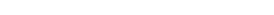is sort of a cycle right?

Now, the symmetry argument starts from this symmetric figure.

We will do the problem for general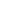.

Central Idea: Let's fix a pair say [ 4 - 5 ], we will see in all the permutations, in how many times, [ 4 - 5 ] can occur.

We will see that there is nothing particular about [ 4 - 5 ], and this is the symmetry argument. Therefore, the number is symmetric along with all such pairs.

Observe, along every such cycle containing [ 4 - 5 ], there are three parameters:

• The position of the [ 4 - 5 ] in which edge of the cycle?
• The permutation of that [ 4 - 5 ], as [ 4 - 5 ] or [ 5 - 4 ].
• The number of arrangements of the remaining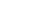numbers.

The number corresponding to the above questions are the following:

•options of position of edge since, there areedges.
• 2! = 2 ways of arranging.
•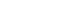ways of arranging the rest of thenumbers.

So, in total [ 4 - 5 ] edge will occur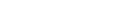times.

By the symmetry argument, every edge [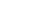], will occurtimes.

Thus, when we sum over all such permutations, we get the following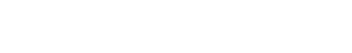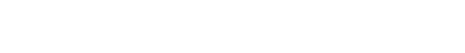Now, there are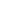permutations in total. So, to take the average, we divide byto get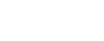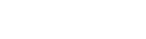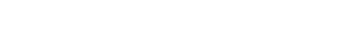#### Edit 1:

One of the readers, Vishal Routh has shared his solution using Conditional Expectation, I am sharing his solution in picture format.

## Video Solution:

This problem is a beautiful and elegant application of basic counting principles, symmetry and double counting principles in combinatorics. This is Problem 2 from ISI MStat 2016 PSB.

## Problem

Determine the average value oftaken over all permutationsof.

## Solution

The problem may seem mind boggling at first, when you will even try to do it for, instead of.

But, in mathematics, symmetry is really intriguing. Let's see how a symmetry argument holds here. It is just by starting to count. Let's see this problem in a geometrical manner.is sort of a cycle right?

Now, the symmetry argument starts from this symmetric figure.

We will do the problem for general.

Central Idea: Let's fix a pair say [ 4 - 5 ], we will see in all the permutations, in how many times, [ 4 - 5 ] can occur.

We will see that there is nothing particular about [ 4 - 5 ], and this is the symmetry argument. Therefore, the number is symmetric along with all such pairs.

Observe, along every such cycle containing [ 4 - 5 ], there are three parameters:

• The position of the [ 4 - 5 ] in which edge of the cycle?
• The permutation of that [ 4 - 5 ], as [ 4 - 5 ] or [ 5 - 4 ].
• The number of arrangements of the remainingnumbers.

The number corresponding to the above questions are the following:

•options of position of edge since, there areedges.
• 2! = 2 ways of arranging.
•ways of arranging the rest of thenumbers.

So, in total [ 4 - 5 ] edge will occurtimes.

By the symmetry argument, every edge [], will occurtimes.

Thus, when we sum over all such permutations, we get the followingNow, there arepermutations in total. So, to take the average, we divide byto get#### Edit 1:

One of the readers, Vishal Routh has shared his solution using Conditional Expectation, I am sharing his solution in picture format.

## Video Solution:

This site uses Akismet to reduce spam. Learn how your comment data is processed.

### Knowledge Partner